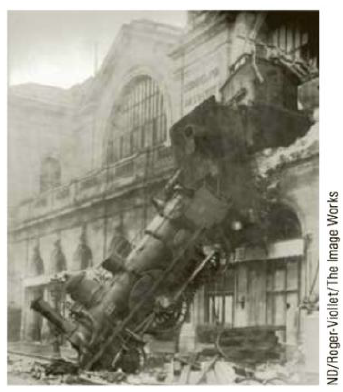Chapter 4, Problem 17CQ

Chapter
Section
Textbook Problem

In Figure 4.4, a locomotive has broken through the wall of a train station. During the collision, what can be said about the force exerted by the locomotive on the wall? (a) The force exerted by the locomotive on the wall was larger than the force the wall could exert on the locomotive. (b) The force exerted by the locomotive on the wall was the same in magnitude as the force exerted by the wall on the locomotive. (c) The force exerted by the locomotive on the wall was less than the force exerted by the wall on the locomotive. (d) The wall cannot be said to “exert” a force; after all, it broke.To determine
The force exerted by the locomotive on the wall.

Explanation

According to Newton’s third law, action and reaction forces are equal in magnitude and opposite in direction. Action and reaction forces act on different objects.

Conclusion:

The locomotive colliding with wall is the action. The wall exerting a force on the locomotive is the reaction. Thus, option (b) is correct.

For the force exerted by the locomotive on the wall to be greater than the force exerted by the wall on the locomotive, an external force should be present along the direction of motion of the locomotive. Thus, option (a) is incorrect...

Still sussing out bartleby?

Check out a sample textbook solution.

See a sample solution

The Solution to Your Study Problems

Bartleby provides explanations to thousands of textbook problems written by our experts, many with advanced degrees!

Get Started

Find more solutions based on key concepts